# Thermal shock behaviour on generalized thermoelastic semi-infinite medium with moving heat source under Green Naghdi-III model

## Biswajit Singh1, Smita Pal (Sarkar)2

1, 2Department of Mathematics, Indian Institute of Engineering Science and Technology (IIEST), Shibpur, Howrah, 711103, West Bengal, India

1Corresponding author

Mathematical Models in Engineering, Vol. 5, Issue 3, 2019, p. 79-89. https://doi.org/10.21595/mme.2019.20904
Received 14 July 2019; received in revised form 13 August 2019; accepted 21 August 2019; published 30 September 2019

Copyright © 2019 Biswajit Singh, et al. This is an open access article distributed under the Creative Commons Attribution License, which permits unrestricted use, distribution, and reproduction in any medium, provided the original work is properly cited.
Editor's Pick
Views 263
Abstract.

The present article deals with the thermal shock response in an isotropic thermoelastic medium with a moving heat source. In this context Green and Naghdi type III model of generalized thermoelasticity theory is considered. The basic equations are expressed as vector-matrix differential equation form. The considered formulation is applied to a semi-infinite solid space. The analytical formulations of the problem in the Laplace transform domain have been solved by eigenvalue approach technique. The inversion of Laplace transform is completed by Zakian method. The variation of the temperature, displacement and stress distributions for different values of time and heat source velocity are shown graphically for two different cases. In the first case, a thermal shock free surface is considered subjected to traction and in the second case the surface is under the influence of time dependent thermal shock. Finally, some comparisons of the results for different time and moving heat source velocity are presented. In presence of moving heat source all the thermophysical quantities have a great significant effect in all the distributions.

Highlights
• An eigenvalue approach method is introduced to obtain the analytical expression of the physical quantities of the problem of generalized thermoelasticity with moving heat source.
• Significant differences of the displacement component, stress and temperature field are seen for different values time and velocity.
• It can be noted that moving heat source velocity has a great expressive effect on the solutions of stress, displacement and temperature field.
• From the figures it is clear that the thermal and mechanical boundary conditions is satisfied which indicates that the analytical results agree with numerical results.

Keywords: generalized thermoelasticity, eigenvalue approach, Laplace transform, vector-matrix differential equation.

#### 1. Introduction

The topic generalized thermoelasticity has increased more consideration of several researchers during last four decades due to its applications in so many fields of applied sciences and mathematics viz. earthquake engineering, nuclear reactor's design, soil dynamics, high energy particle accelerators, etc. The objective of this theory is to succeed in the shortcomings that are inherent in the classical thermoelasticity theory suggested by Biot . In this theory the heat equation is of parabolic type. The infinite speed of thermoelastic disturbance is inherent in that theory and it is unrealistic from physical point of view. To resolve this problem, the classical thermoelasticity theory has been generalized so that a finite speed of thermoelastic disturbance is admitted. In generalized thermoelasticity, the parabolic type heat equation is replaced by hyperbolic type heat equation supported by examinations which display the real existence of wave type heat transportation in solids, known as the second sound effect. The first is because of Cattaneo  and Vernotte  who proposed generalized version of Fourier’s law to obtain hyperbolic heat conduction equation by introducing a relaxation time, named hereafter C-V model.

The first generalization of the classical theory of thermoelasticity, determined by Lord and Shulman , included one relaxation time parameter in Fourier’s law of heat conduction equation. It involved a heat transfer equation of hyperbolic nature declaring finite speed of thermal signals. The temperature-rate-dependent theory (TRDTE) of thermo-elasticity as proposed by Green and Lindsay , called G-L theory, involved two relaxation time parameters. This hypothesis achieved a modified variety of the constitutive equations in the coupled theory of thermoelasticty. Suhubi  freely found explicit type of constitutive equations. Consequently, a few works [7, 8] based on these generalized models had been examined. Hetnarski and Ignaczak  developed low-temperature thermoelasticity dependent on the third generalization of the coupled theory and classified it by a system of non-linear field equations. Heat conduction equation for low-temperature non-linear model estimates that the wave like-thermal signals were supposed to grip at low temperatures. Kosinski et al. [10, 11] had examined Soliton-like waves in a low-temperature non-linear thermoelastic solid. Green and Naghdi [12, 13] formulated the fourth generalization to the theory of coupled thermoelasticity (known as G-N theory) which was concerned without energy dissipation. This theory pursues the Fourier law of heat conduction and proves that heat propagates with finite speed. Chandrasekharaiah et al.  had contemplated collaborations with G-N theory without energy dissipation (TEWOED) in an unbounded medium with spherical cavity body. Roy Choudhuri et al.  considered propagation of wave with G-N theory without energy dissipation in rotating elastic medium. Numerous problems involving to the G-N theory without energy dissipation other than generalized thermoelasticity were studied [16-19].

Several authors including Gutfield and Netherchot , Taylor et al.  conducted experiments with various solid bodies and showed that heat pulses do not propagate at infinite speed. He and Cao  considered generalized magneto thermoelastic problem subjected to moving heat source. Also, Chandrasekharaiah and Srinath  studied the thermolastic interaction without energy dissipation due to a point heat source and line heat source.

Some applications of magneto thermorelasticity using eigenvalue approach technique were examined in the literatures [24, 25]. Das and Bhakata  proposed eigenfunction expansion technique to the solution of simultaneous equations and its application in mechanics. Baksi et al.  examined magneto-thermoelastic interactions with heat sources and thermal relaxation in a three dimensional unbounded rotating elastic medium. Abbas [28-33]and his colleagues solved different types of problem by eigenvalue approach method which was developed by Das et al.  in Laplace transform domain. Baksi et al.  and Sinha et al.  solved various types of problems considering the effect of rotation and relaxation time in generalized thermoelasticity using eigenvalue approach method. Lahiri et al.  used matrix method of solution of coupled differential equation and showed its applications in generalized thermoelasticity.

In this present article, a problem of generalized thermoelasticity subjected to a moving heat source distributed over a plane area in an unbounded isotropic medium is considered. The governing equation of the problem is solved by eigenvalue approach technique developed by Das et al. . The analytical solutions are found for displacement, temperature and stress in the Laplace transform domain. The inversion of Laplace transform has been computed using efficient computer programming. Finally, the resulting quantities to study the effect of the moving heat source for copper material are derived numerically and presented graphically for two different cases.

#### 2. The governing equation

In absence of body force equations of motion take the following form :

(1)
${\sigma }_{ij,j}=\rho {\stackrel{¨}{u}}_{i}.$

The heat conduction is of the form:

(2)
${K}^{*}{\nabla }^{2}T+K{\nabla }^{2}\stackrel{˙}{T}=\frac{\partial }{\partial t}\left(\rho {c}_{e}\stackrel{˙}{T}+\gamma {T}_{0}\stackrel{˙}{e}-Q\right).$

The constitutive equation is given by:

(3)
${\sigma }_{ij}=2\mu {e}_{ij}+\left[\lambda e-\gamma \left(T-{T}_{0}\right)\right]{\delta }_{ij}.$

Special cases:

If we take $K=0$ then the problem will be reduced to Green-Naghdi II theory (GN-II).

If we take ${K}^{*}=\text{0}$ then the problem will be reduced to Green-Naghdi I theory (GN-I).

The displacement u and the temperature field $T$ can be written for one- dimensional problem in the following form: $u=u\left(x,t\right)$, $v=0$, $w=0$, $T=T\left(x,t\right)$.

Thus Eqs. (1), (2), (3) take the following form:

(4)
$\left(\lambda +2\mu \right)\frac{{\partial }^{2}u}{\partial {x}^{2}}-\gamma \frac{\partial T}{\partial x}=\rho \frac{{\partial }^{2}u}{\partial {t}^{2}},$
(5)
${K}^{*}\frac{{\partial }^{2}T}{\partial {x}^{2}}+K\frac{{\partial }^{3}T}{\partial t\partial {x}^{2}}=\frac{\partial }{\partial t}\left(\rho {c}_{e}\frac{\partial T}{\partial t}+\gamma {T}_{0}\frac{{\partial }^{2}u}{\partial t\partial x}-Q\right),$
(6)
${\sigma }_{xx}=\left(\lambda +2\mu \right)\frac{\partial u}{\partial x}-\gamma \left(T-{T}_{0}\right).$

Introducing the non-dimensional variables:

(7)

where:

For simplicity dropping *, Eqs. (4) -(6) take the non-dimensional form as:

(8)
$\frac{{\partial }^{2}u}{\partial {x}^{2}}-\frac{\partial T}{\partial x}=\frac{{\partial }^{2}u}{\partial {t}^{2}},$
(9)
${\epsilon }_{1}\frac{{\partial }^{2}T}{\partial {x}^{2}}+\frac{{\partial }^{3}T}{\partial t\partial {x}^{2}}=\frac{\partial }{\partial t}\left(\frac{\partial T}{\partial t}+{\epsilon }_{2}\frac{{\partial }^{2}u}{\partial t\partial x}-Q\right),$
(10)
${\sigma }_{xx}=\frac{\partial u}{\partial x}-T.$

The non-dimensional form of the moving heat source is taken in the following form :

(11)
$Q={Q}_{0}\delta \left(x-vt\right),$

where ${Q}_{0}$ is constant and $\delta$ is the delta function and $v$ is the heat source velocity.

#### 3. Initial and boundary conditions

For preceding description, we assume that the medium is initially at rest i.e. at time $t=$ 0, the displacement component and temperature along with their derivatives with respect to t are zero. So the following initial conditions hold:

(12)

Now the boundary conditions at $x=\text{0}$ are taken as.

Case (I):

(13)

where, $H\left(t\right)$ indicates the Heaviside function.

Case (II).

Thermal boundary condition:

Mechanical boundary condition:

(14)

where, $F\left(t\right)={T}^{*}H\left(t\right)$ and ${T}^{*}$ denotes the constant temperature.

#### 4. Laplace transform domains

We define the Laplace transformation on the function $f\left(t\right)$ as follows:

(15)
$\stackrel{-}{f}\left(s\right)=L\left[f\left(t\right)\right]={\int }_{0}^{\infty }f\left(t\right){e}^{-st}dt.$

Applying the Laplace transform under initial conditions to Eqs. (8-10) and (13), (14) we get:

(16)
$\frac{{d}^{2}\stackrel{-}{u}}{d{x}^{2}}-\frac{d\stackrel{-}{T}}{dx}={s}^{2}\stackrel{-}{u},$
(17)
$\left({\epsilon }_{1}+s\right)\frac{{d}^{2}\stackrel{-}{T}}{d{x}^{2}}={s}^{2}\stackrel{-}{T}+{s}^{2}{\epsilon }_{2}\frac{d\stackrel{-}{u}}{dx}-{Q}_{0}\frac{s}{v}{e}^{-\frac{sx}{v}},$
(18)
${\stackrel{-}{\sigma }}_{xx}=\frac{d\stackrel{-}{u}}{dx}-\stackrel{-}{T},$
(19)
(20)

#### 5. Formulation of the vector- matrix differential equation

The Eqs. (16) and (17) are expressed in the vector-matrix differential form as follows:

(21)
$\frac{d}{dx}\left[\begin{array}{l}\stackrel{-}{u}\\ \begin{array}{l}\stackrel{-}{T}\\ {\stackrel{-}{u}}^{\text{'}}\end{array}\\ {\stackrel{-}{T}}^{\text{'}}\end{array}\right]=\left[\begin{array}{cccc}0& 0& 1& 0\\ 0& 0& 0& 1\\ {M}_{31}& 0& 0& {M}_{34}\\ 0& {M}_{42}& {M}_{43}& 0\end{array}\right]\left[\begin{array}{l}\stackrel{-}{u}\\ \begin{array}{c}\stackrel{-}{T}\\ {\stackrel{-}{u}}^{\text{'}}\end{array}\\ {\stackrel{-}{T}}^{\text{'}}\end{array}\right]+\left[\begin{array}{c}0\\ 0\\ 0\\ m{e}^{-sx/v}\end{array}\right],$

where:

(22)

Eq. (21) can be compactly written as:

(23)
$\frac{d\stackrel{-}{V}}{dx}=\stackrel{-}{A}\left(s\right)\stackrel{-}{V}+\stackrel{-}{f},$

where:

#### 6. Solution of the vector matrix differential equation

The characteristic equation of the matrix $A$ is given by :

(24)
${\eta }^{4}-\left({M}_{31}+{M}_{42}+{M}_{34}{M}_{43}\right){\eta }^{2}+{M}_{31}{M}_{42}=0.$

The eigenvalues of the matrix $\stackrel{-}{A}\left(s\right)$, which are also roots of the characteristic Eq. (24), take the form:

(25)

The right eigenvector corresponding to the eigenvalue $\eta$ can be written as:

(26)

From Eqs. (25) and (26), the eigenvector ${X}_{i}$ corresponding to the eigenvalue $\eta ={\eta }_{i}\text{,}$$i=$ 1, 2, 3, 4 can be easily calculated. The following notations will be used:

(27)

Similarly, the left eigenvector , corresponding to the eigenvalue $\eta$ can be calculated as:

(28)

From Eqs. (25) and (28), we can easily obtain the eigenvector ${Y}_{i}$ corresponding to the eigenvalue $\eta ={\eta }_{i}$, $i=$ 1, 2, 3, 4 we use the notation as:

(29)

Thus, the complementary solution of the Eq. (21) can be written as follows:

(30)
${\stackrel{\to }{V}}_{c}=\sum _{k=1}^{4}{A}_{k}{\stackrel{\to }{X}}_{k}{e}^{{\eta }_{k}x}={A}_{2}{\left[\stackrel{\to }{X}\right]}_{\eta =-{\eta }_{1}}{e}^{-{\eta }_{1}x}+{A}_{4}{\left[\stackrel{\to }{X}\right]}_{\eta =-{\eta }_{2}}{e}^{-{\eta }_{2}x}.$

Since the components of the vector $\stackrel{\to }{V}$ are all finite, when $x\to \infty$ we get ${A}_{1}$, ${A}_{3}\to 0.$ Therefore, the particular solution ${\stackrel{\to }{V}}_{p}$ should be in the form:

(31)

where:

(32)
(33)

Thus, the general solution of the non-homogeneous system Eq. (23) is:

(34)
$\stackrel{\to }{V}\left(x,s\right)={\stackrel{\to }{V}}_{c}+{\stackrel{\to }{V}}_{p}.$

Thus, the expressions of displacement, temperature and stress can be written from Eq. (34) as:

(35)
$\stackrel{-}{u}\left(x,s\right)=-{A}_{2}{M}_{34}{\eta }_{1}{e}^{-{\eta }_{1}x}-{A}_{4}{M}_{34}{\eta }_{2}{e}^{-{\eta }_{2}x}-{\eta }_{1}{a}_{2}\left(x\right){e}^{-{\eta }_{1}x}-{\eta }_{2}{a}_{4}\left(x\right){e}^{-{\eta }_{2}x},$
(36)
$\stackrel{-}{T}\left(x,s\right)={A}_{2}\left({{\eta }_{1}}^{2}-{M}_{31}\right){e}^{-{\eta }_{1}x}+{A}_{4}\left({{\eta }_{2}}^{2}-{M}_{31}\right){e}^{-{\eta }_{2}x}$
(37)
${\overline{\sigma }}_{xx}\left(x,s\right)={A}_{2}{M}_{34}{\eta }_{1}^{2}{e}^{-{\eta }_{1}x}+{A}_{4}{M}_{34}{\eta }_{2}^{2}{e}^{-{\eta }_{2}x}$

With the help of the boundary conditions, the constants ${A}_{2}$, ${A}_{4}$ can be obtained for case (I) and case (II).

#### 7. Numerical inversion

For the final solution of temperature, displacement and stress distribution in the time domain, the Zakian  method for the inversion of Laplace Transform has been applied.

This method $f\left(t\right)$ is computed as a sum of weighted evaluations of $F\left(s\right)$:

$f\left(t\right)=\sum _{i=1}^{N}{K}_{i}F\left({S}_{i}\right),$

where the values of ${K}_{i}$, ${S}_{i}$ and $N$ are dictated by a particular method. A significant feature of the derivation is the specification that the time function can be related to a finite series of exponential functions:

$\sum _{i=1}^{N}{K}_{i}{e}^{{\alpha }_{i}t}.$

The significance of this specification is that Zakian’s Algorithm is very accurate for overdamped and slightly underdamped systems. But it is not accurate for systems with prolonged oscillations.

Given $F\left(s\right)$ and a value of time t, the following equation implements Zakian’s Algorithm and allows us to calculate the numerical value of $f\left(t\right)$:

$f\left(t\right)=\frac{2}{t}\sum _{i=1}^{5}Real\left({K}_{i}F\left(\frac{{\alpha }_{i}}{t}\right)\right).$

Table 1. Values of complex constant for ${\alpha }_{i}$ and ${K}_{i}$ as in 

 $i$ ${\alpha }_{i}$ ${K}_{i}$ 1 12.83767675+$i$1.666063445 –36902.0821+$i$196990.426 2 12.22613209+$i$5.012718792 61277.0252–$i$95408.6255 3 10.9343031+$i$8.40967312 –28916.5629+$i$18169.1853 4 8.77643472+$i$11.9218539 4655.36114–$i$1.90152864 5 5.22545336+$i$15.7295290 –-118.741401–$i$141.303691

Zakian’s Algorithm is simple to implement and computes quickly.

#### 8. Numerical results and discussion

For the purpose of numerical simulations, the copper material was chosen, the physical data for which are given in Table 2.

Table 2. Material constants

 Symbol Numerical value Units $\lambda$ 7.76×1010 (kg)(m)-1(s)-1 $\mu$ 3.86×1010 (kg)(m)-1(s)-2 ${T}_{0}$ 293 K $K$ 3.86×102 (kg)(m)(K)-1(s)-3 ${c}_{e}$ 3.831×102 (m)2(K)-1(s)-2 $\rho$ 8.954×103 (kg)(m)-2 ${\alpha }_{t}$ 1.78×10-6 (K)-1

The other constants are specified as ${Q}_{0}=$ 7, ${T}^{\mathrm{*}}=$ 0.6.

Using above data to study the characteristics of displacement, stresses and temperature, we have drawn several figures, Figs. 1-6 for case I and Figs. 7-12 for case II for different values of time and velocity of the moving heat source with distance $x$, and here the velocity of moving heat source and time has a great effect on all distributions.

Case-(I): Figs. 1-3 depicts the effect of Temperature ($T$), displacement ($u$) and stress $\left(\sigma \right)$ against distance for different values of time (0.003, 0.006, 0.009) where velocity of moving heat source, $v=$ 1.5 remains fixed. Figs. 4-6 exhibits the consequence of Temperature ($T$), displacement ($u$) and stress $\sigma$ with distance for different values of velocity (1.6, 1.8, 2.0) where ($t=$ 0.002) remains constant. From Fig. 1 it is seen that magnitude of the temperature decreases with the increase of time and components of temperature obtain its maximum value near 0.6 and then it decreases to zero for all values of t. Figs. 2, 4, 5 it is observed that the magnitude of the absolute displacement, absolute temperature increases with the increase of time and velocity. From Fig. 3 we see that absolute value of stress increase with increase of time. Fig. 6 shows that when velocity increases then the magnitude of the stress decreases and stress component will decrease its maximum near 2. In all the Figs. 1, 3 and Figs. 4, 6 stress values always initiate from one and end with zero value and magnitude of the temperature starts from zero value and terminate with zero value. These two components also satisfy the physical boundary conditions of the considered problem.

Fig. 1. Distribution of temperature against distance for distinct values of $t$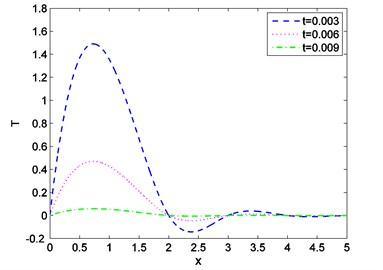Fig. 2. Distribution of displacement against distance for various values of $t$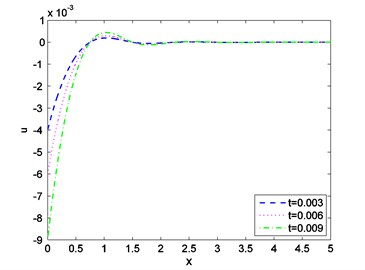Fig. 3. Distribution of stress against distance for various values of $t$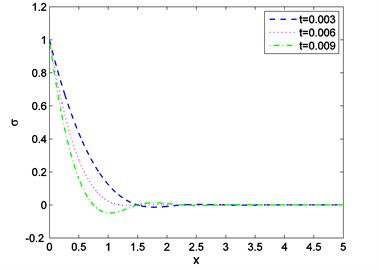Fig. 4. Distribution of temperature against distance for various values of $v$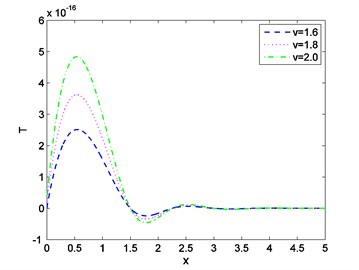Fig. 5. Distribution of displacement against distance for various values of $v$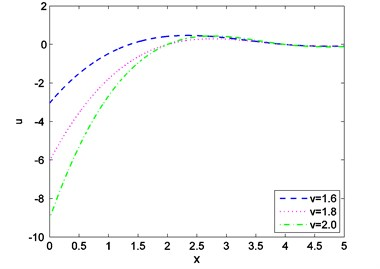Fig. 6. Distribution of stress against distance for various values of $v$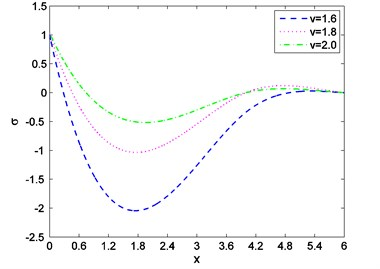Fig. 7. Distribution of temperature against distance for various values of $t$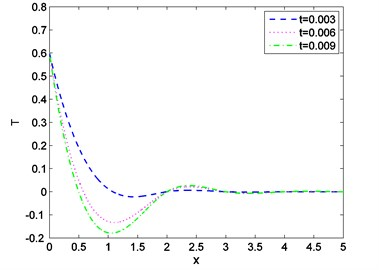Fig. 8. Distribution of displacement against distance for various values of $t$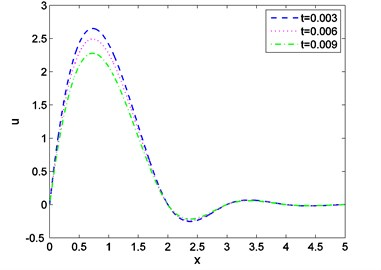Fig. 9. Distribution of stress against distance for various values of $t$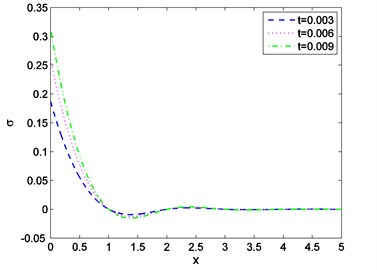Fig. 10. Distribution of temperature against distance for various values of $v$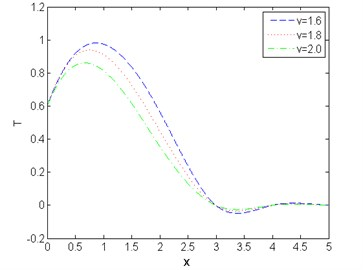Fig. 11. Distribution of displacement with distance for various values of $v$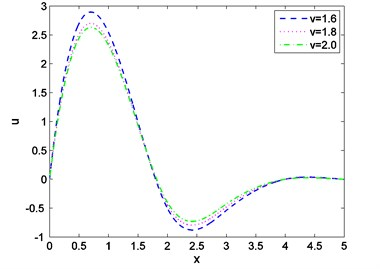Fig. 12. Distribution of stress with distance for various values of $v$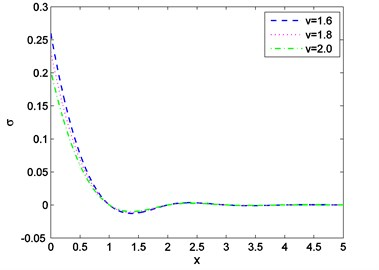Case-II: That the absolute value of the temperature distribution increases with increase of time is observed from Fig. 7. Fig. 8 shows that magnitude of displacement decrease with increase of time. From Fig. 9 we observed that distribution of stress increases with the increase of time. From Figs. 10, 11, 12 it is seen that magnitude of displacement, stress and temperature components decrease with increase of velocity of heat source. Finally, all these figures always obey the boundary conditions for this case.

#### 9. Conclusions

In this work, the magnitude of the displacement, temperature and stress have been studied for two cases. In the first case, the surface of the half space 0 is subjected to a traction and thermal shock free whereas in the second case, the surface is subjected to a time dependent thermal shock. Analytical expressions for stress, temperature and displacement in the material have been derived. Eigenvalue approach method in Laplace transform domain furnishes good approximation of the solution. It can be noted that moving heat source velocity has a great expressive effect on the solutions of stress, displacement and temperature field.

The results presented in this paper may be helpful for researchers who are working on mathematical physics, mathematical physics, thermodynamics with low temperatures as well as on the development of the hyperbolic thermoelasticity theory.

1. Biot M. A. Thermoelasticity and irreversible thermodynamics. Journal of Applied Physics, Vol. 27, 1956, p. 240-253. [Publisher]
2. Cattaneo C. Sur une former de l’equation de la chaleurelinant le paradoxed’une propagation instance. Comptes Rendus de l’Académie des Sciences, Vol. 247, 1958, p. 431-432. [Search CrossRef]
3. Vernotte M. P. Les paradoxes de la theorie continue de l’equation de la chaleur. Comptes Rendus de l’Académie des Sciences, Vol. 246, 1958, p. 3154-3155. [Search CrossRef]
4. Lord H. W., Shulman Y. A generalized dynamic theory of thermoelasticity. Journal of the Mechanics and Physics of Solids, Vol. 15, 1967, p. 299-309. [Publisher]
5. Green A. E., Lindsay K. A. Thermoelasticity. Journal of Elasticity, Vol. 2, Issue 1, 1972, p. 1-7. [Publisher]
6. Suhubi E. S. Thermoelastic Solids. A. C. Eringen Continuum Physics, Part 2, Academic Press, New York, 1975. [Publisher]
7. Wang J., Dhaliwal R. S., Rokne J. G. Fundamental solutions of the generalized thermo-elastic equations. Journal of Thermal Stresses, Vol. 16, 1993, p. 135-161. [Publisher]
8. Chandrasekharaiah D. S. Wave propagation in thermo-elastic half space. Indian Journal of Pure and Applied Mathematics, Vol. 12, 1981, p. 226-241. [Search CrossRef]
9. Hetnarski R. B., Ignaczak J. Soliton-like waves in a low-temperature non-linear thermoelastic solid. International Journal of Engineering Science, Vol. 34, 1996, p. 1767-1787. [Publisher]
10. Kosinski W. Elastic Waves in the Presence of a New Temperature Scale in Elastic Wave Propagation. Elsevier, New York, 1989, p. 629-634. [Publisher]
11. Kosinski W., Cimmelli V. A. Gradient generalization to inertial state variables and a theory of super fluidity. Journal of Theoretical Applied Mechanics, Vol. 35, 1997, p. 763-779. [Search CrossRef]
12. Green A. E., Naghdi P. M. On undamped heat waves in an elastic solid, Journal of Thermal Stresses, Vol. 15, 1992, p. 253-264. [Publisher]
13. Green A. E., Naghdi P. M. Thermoelasticity without energy dissipation. Journal of Elasticity, Vol. 31, Issue 89, 1993, p. 208-1993. [Search CrossRef]
14. Chandrasekharaiah D. S., Murthy H. N. Thermoelastic interactions in an unbounded body with a special Cavity. Journal of Thermal Stresses, Vol. 16, 1993, p. 55-70. [Publisher]
15. Roy Choudhuri S. K., Bandyopadhyay N. Thermoelastic wave propagation in rotating elastic medium without energy dissipation. International Journal of Mathematics and Mathematical Sciences, Vol. 2005, Issue 1, 2005, p. 99-107. [Publisher]
16. Kumar R., Chawla V. Wave propagation at the boundary surface of elastic layer overlaying a thermoelastic without energy dissipation half-space. Journal of Solid Mechanics, Vol. 2, Issue 4, 2010, p. 363-375. [Search CrossRef]
17. Dhaliwal R. S., Rokne J. G. One-dimensional generalized thermoelastic problem for a half-space. Journal of Thermal Stresses, Vol. 11, 1988, p. 257-271. [Publisher]
18. Chandrasekharaiah D. S., Srinath K. S. One-dimensional waves in a thermoelastic half-space without energy dissipation. International Journal of Engineering Science, Vol. 34, Issue 13, 1996, p. 1447-1455. [Publisher]
19. Abbas I. A. A GN model for thermoelastic interaction in an unbounded fiber-reinforced anisotropic medium with a circular hole. Applied Mathematics Letters, Vol. 26, Issue 2, 2013, p. 232-239. [Publisher]
20. Gutfeld R. J., Nethercot A. H. Temperature dependence of heat- pulse propagation in Sapphire. Physical Review Letters, Vol. 17, 1966, p. 868-871. [Publisher]
21. Taylor B., Maris H. J., Elbaum C. Phonon focusing in solids. Physical Review Letters, Vol. 23, 1969, p. 416-419. [Publisher]
22. Cao L. A problem of generalized magneto-thermoelastic thin slim strip subjected to a moving heat source. Mathematical and Computer Modeling, Vol. 49, Issues 7-8, 2009, p. 1710-1720. [Publisher]
23. Chandrasekharaiah D. S., Srinath K. S. Thermoelastic interactions without energy dissipation due to point heat source. Journal of Elasticity, Vol. 50, Issue 2, 1998, p. 97-108. [Publisher]
24. Das B., Lahiri A. One dimensional generalized magneto thermoelastic problem for a half space. Proceedings of the World Congress on Engineering, 2012. [Search CrossRef]
25. Sarkar N., Lahiri A. Electromagneto thermoelastic interactions in an orthotropic slab with two thermal relaxation times. Computational Mathematics Modelling, Vol. 23, Issue 4, 2012, p. 461-477. [Publisher]
26. Das N. C., Bhakata P. C. Eigen function expansion method to the solution of simultaneous equations and its application in mechanics. Mechanics Research Communications, Vol. 12, 1985, p. 19-29. [Publisher]
27. Baksi A., Bera R. K., Debnath L. A study of magneto-thermoelastic problems with thermal relaxation and heat sources in a three dimensional infinite rotating elastic medium. International Journal of Engineering Science, Vol. 43, Issues 19-20, 2005, p. 1419-1434. [Publisher]
28. Abbas I. A., Abo Dahab S.-M. On the numerical solution of thermal shock problem for generalized magneto thermoelasticity for an infinitely long annular cylinder with variable thermal conductivity. Journal of Computational and Theoretical Nanoscience, Vol. 11, Issue 3, 2014, p. 607-618. [Publisher]
29. Abbas I. A. A GN model based upon two-temperature generalized thermoelastic theory in an unbounded medium with a spherical cavity. Applied Mathematics and Computation, Vol. 245, 2014, p. 108-115. [Publisher]
30. Abas I. A., Abd-Alla, A. N., Othman M. I. A Generalized magneto-thermoelasticity in a fiber- reinforced anisotropic half-space. International Journal of Thermophysics, Vol. 32, Issue 5, 2011, p. 1071-1085. [Publisher]
31. Abbas I. A. Eigenvalue approach for an unbounded medium with a spherical cavity based upon two temperatures generalized thermoelastic theory. Journal of Mechanical Science and Technology, Vol. 28, Issue 10, 2014, p. 4193-4198. [Publisher]
32. Abbas I. A. Eigenvalue approach in three-dimensional generalized thermoelastic interactions with temperature dependent material properties. Computers and Mathematics with Applications, Vol. 68, Issue 12, 2014, p. 2036-2056. [Publisher]
33. Abbas I. A. Generalized magneto thermoelastic interaction in a fiber-reinforced anisotropic hollow cylinder. International Journal of Thermophysics, Vol. 33, Issue 3, 2012, p. 567-579. [Publisher]
34. Baksi A., Bera R. K., Debnath L. Eigenvalue approach the effect of rotation and relaxation time in two dimensional problems of generalized thermoelasticity. International Journal of Engineering Science, Vol. 42, 2004, p. 1573-1585. [Publisher]
35. Sinha M., Bera R. K. Eigenvalue approach the effect of rotation and relaxation time in generalized thermoelasticity. Computers and Mathematics with Applications, Vol. 46, 2003, p. 783-792. [Publisher]
36. Lahiri A., Das N. C., Sarkar S., Das M. Matrix method of solution of coupled differential equations and its application to generalized thermoelasticity. Bulletin of the Calcutta Mathematical Society, Vol. 101, 2009, p. 571-590. [Search CrossRef]
37. Zakian V. Optimization of numerical inversion of Laplace transforms. Electronics Letters, Vol. 6, 1970, p. 667-679. [Search CrossRef]
38. Das N. C., Lahiri A., Giri R. R. Eigenvalue approach to generalized thermoelasticity. Indian Journal of Pure and Applied Mathematics, Vol. 28, Issue 12, 1997, p. 1573-1594. [Search CrossRef]
39. Othman M. I. A., Abbas I. A. Generalized thermoelasticity of Thermal-Shock problem in a non-homogeneous isotropic hollow cylinder with energy dissipation. International Journal of Thermophysics, Vol. 33, Issue 5, 2012, p. 913-923. [Publisher]
40. Tianhu H., Ying G. A one-dimensional thermoelastic problem due to a moving heat source under fractional order theory of thermoelasticity. Advances in Materials Science and Engineering, Vol. 2014, 2014, p. 510205. [Search CrossRef]

#### Cited By

 Physica Scripta E Awwad, Ahmed E Abouelregal, Doaa Atta, Hamid M Sedighi 2022 Mechanics Based Design of Structures and Machines Biswajit Singh, Smita Pal (Sarkar) 2022 Waves in Random and Complex Media Saroj Mandal, Biswajit Singh, Smita Pal(Sarkar) 2022 Waves in Random and Complex Media Biswajit Singh, Smita Pal (Sarkar) 2022 Archive of Applied Mechanics Biswajit Singh, Smita Pal Sarkar 2021 Journal of Thermal Stresses Biswajit Singh, Smita Pal (Sarkar), Krishnendu Barman 2020 Journal of Heat Transfer Biswajit Singh, Indranil Sarkar, Smita Pal (Sarkar) 2020 Results in Physics Ahmed E. Abouelregal, Shao-Wen Yao, Hijaz Ahmad 2020